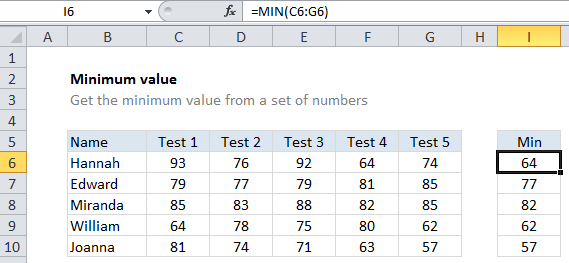## Excel Office

Excel How Tos, Tutorials, Tips & Tricks, Shortcuts

# Minimum value in Excel

This tutorial shows how to calculate Minimum value in Excel using the example below;

## Formula

`=MIN(range)`## Explanation

To get the minimum value from a set of numbers, use the MIN function.

Worked Example:   How to get relative row numbers in a range in Excel

In the example shown, the formula in cell I6 is:

`=MIN(C6:G6)`

The MIN function is fully automatic. It accepts one or more arguments, which can be numbers or cell references that point to numbers, then returns the minimum value.